Home > GC > Chapter 8 > Lesson 8.3.1 > Problem8-86

8-86.
1. For each triangle below, find the value of x, if possible. Name which triangle tool you used. If the triangle cannot exist, explain why. Homework Help ✎

1.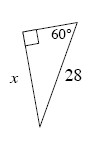2.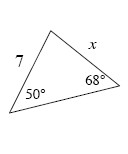3.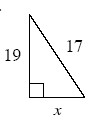4.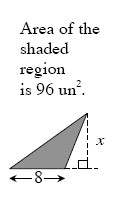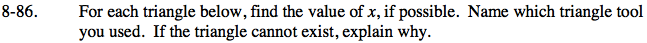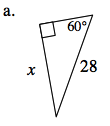What special kind of triangle is this? How can this help you find x?

$x=14\sqrt{3}$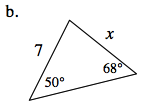Use the Law of Sines to solve for x.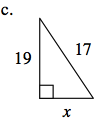Use the Pythagorean Theorem to find x.

There is no solution, because the hypotenuse must be the longest side.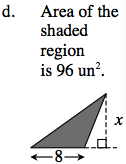What is the formula for the area of a triangle?